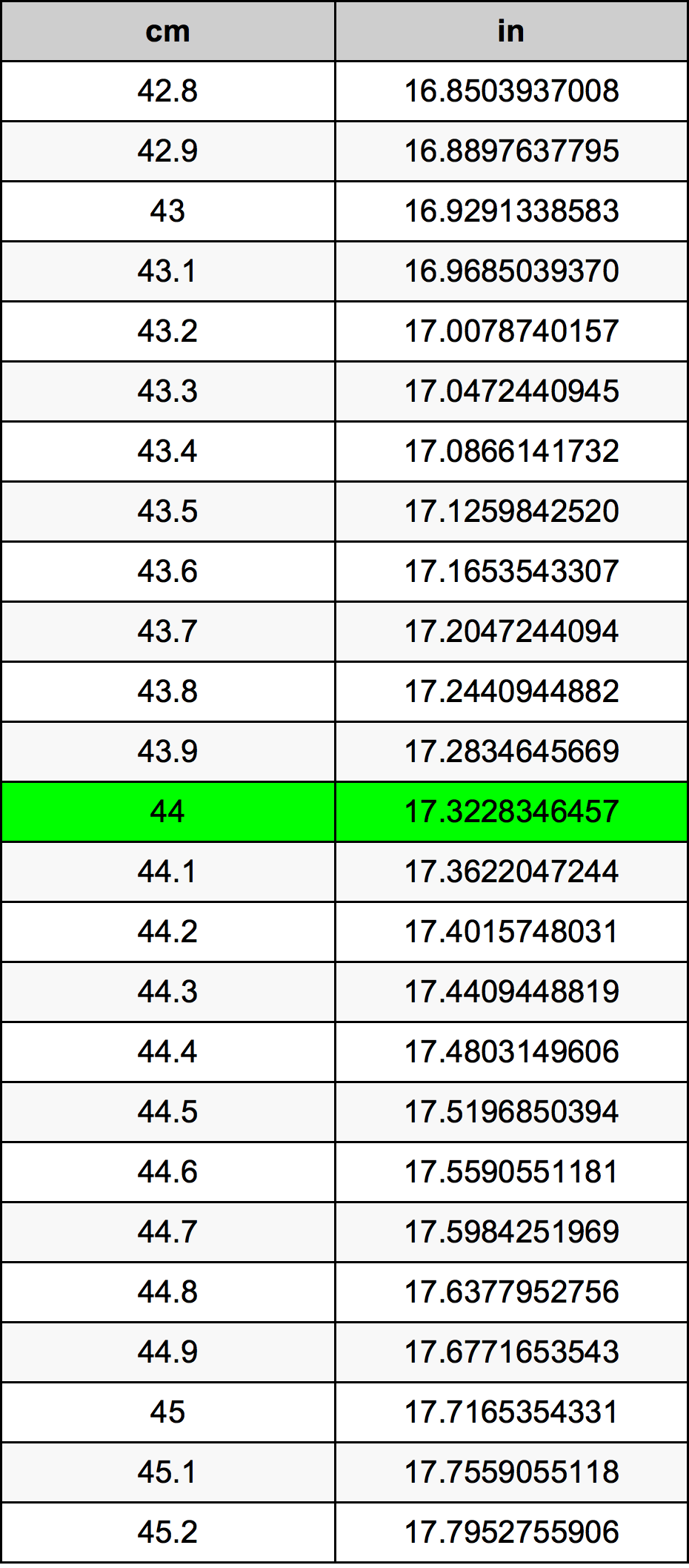Cm To Inches

# 44 cm to in44 Centimeters to Inches

cm
=
in

## How to convert 44 centimeters to inches?

 44 cm * 0.3937007874 in = 17.3228346457 in 1 cm
A common question is How many centimeter in 44 inch? And the answer is 111.76 cm in 44 in. Likewise the question how many inch in 44 centimeter has the answer of 17.3228346457 in in 44 cm.

## How much are 44 centimeters in inches?

44 centimeters equal 17.3228346457 inches (44cm = 17.3228346457in). Converting 44 cm to in is easy. Simply use our calculator above, or apply the formula to change the length 44 cm to in.

## Convert 44 cm to common lengths

UnitLengths
Nanometer440000000.0 nm
Micrometer440000.0 µm
Millimeter440.0 mm
Centimeter44.0 cm
Inch17.3228346457 in
Foot1.4435695538 ft
Yard0.4811898513 yd
Meter0.44 m
Kilometer0.00044 km
Mile0.0002734033 mi
Nautical mile0.000237581 nmi

## What is 44 centimeters in in?

To convert 44 cm to in multiply the length in centimeters by 0.3937007874. The 44 cm in in formula is [in] = 44 * 0.3937007874. Thus, for 44 centimeters in inch we get 17.3228346457 in.

## 44 Centimeter Conversion Table## Alternative spelling

44 Centimeters to Inch, 44 Centimeters in Inch, 44 Centimeter to in, 44 Centimeter in in, 44 cm to Inch, 44 cm in Inch, 44 cm to in, 44 cm in in, 44 Centimeter to Inches, 44 Centimeter in Inches, 44 Centimeter to Inch, 44 Centimeter in Inch, 44 cm to Inches, 44 cm in Inches